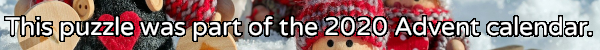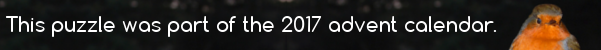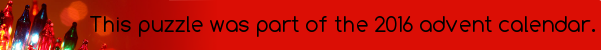mscroggs.co.uk
mscroggs.co.uksubscribe

# Puzzles

## 20 December18 can be written as the sum of 3 consecutive (strictly) positive integers: 5 + 6 + 7.
18 can also be written as the sum of 4 consecutive (strictly) positive integers: 3 + 4 + 5 + 6.
18 is in fact the smallest number that can be written as the sum of both 3 and 4 consecutive (strictly) positive integers.
Today's number is the smallest number that can be written as the sum of both 12 and 13 consecutive (strictly) positive integers.
Tags: numbers, sums

## 24 DecemberThere are six 3-digit numbers with the property that the sum of their digits is equal to the product of their digits. Today's number is the largest of these numbers.

## 6 DecemberNoel's grandchildren were in born in November in consecutive years. Each year for Christmas, Noel gives each of his grandchildren their age in pounds.
Last year, Noel gave his grandchildren a total of £208. How much will he give them in total this year?

## 20 DecemberWhat is the largest number that cannot be written in the form $$10a+27b$$, where $$a$$ and $$b$$ are nonnegative integers (ie $$a$$ and $$b$$ can be 0, 1, 2, 3, ...)?

## Square pairs

Source: Maths Jam
Can you order the integers 1 to 16 so that every pair of adjacent numbers adds to a square number?
For which other numbers $$n$$ is it possible to order the integers 1 to $$n$$ in such a way?

## 14 DecemberIn July, I posted the Combining Multiples puzzle.
Today's number is the largest number that cannot be written in the form $$27a+17b$$, where $$a$$ and $$b$$ are positive integers (or 0).

## Combining multiples

In each of these questions, positive integers should be taken to include 0.
1. What is the largest number that cannot be written in the form $$3a+5b$$, where $$a$$ and $$b$$ are positive integers?
2. What is the largest number that cannot be written in the form $$3a+7b$$, where $$a$$ and $$b$$ are positive integers?
3. What is the largest number that cannot be written in the form $$10a+11b$$, where $$a$$ and $$b$$ are positive integers?
4. Given $$n$$ and $$m$$, what is the largest number that cannot be written in the form $$na+mb$$, where $$a$$ and $$b$$ are positive integers?

## Subsum

1) In a set of three integers, will there always be two integers whose sum is even?
2) How many integers must there be in a set so that there will always be three integers in the set whose sum is a multiple of 3?
3) How many integers must there be in a set so that there will always be four integers in the set whose sum is even?
4) How many integers must there be in a set so that there will always be three integers in the set whose sum is even?

## Archive

Show me a random puzzle
▼ show ▼